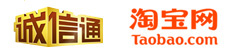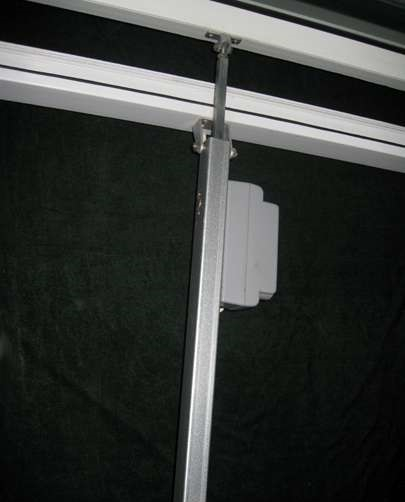## 电动开窗器的力学计算方式

1. 窗的重量计算：
W10使用6mm+9A+6mm+1.14PVB+6mm双层夹胶中空玻璃材质，窗重如下：
W11、W12、W10、W4使用8mm+12A+8mm 双层中空玻璃材质，窗重如下：
G=m*g G为窗扇的重力;m为窗扇的质量;g为重力加速度
m=ρ*V*1.15 ρ为玻璃材质的密度（单位:g/cm3或kg/m3);1.15为窗扇铝（Al)合金(熔点660℃)(alloy)框相对玻璃质量的系数
VW10=0.028m3 VW11、W4=0.025m3 VW12=0.014m3 V为玻璃的体积（volume);
GW10=2.56×103Kg/m3×0.028m3×1.15×10m2/s =824N
GW11、W4=2.56×103Kg/m3×0.025m3×1.15×10m2/s =736N
GW12=2.56×103Kg/m3×0.014m3×1.15×10m2/s =412N

2. 风(雪)载荷计算：
f风(雪)=P*S P按上海八级风压（wind pressure）30Kg/m2、雪压按20Kg/m2;S为风正面作用在窗扇上的面积
f雪W10=312N;f风W11、W4=468N;f风W12=254N
W10窗户根据开窗(windowing )器选型经验（experience)公式得(见下图计算公式)：
F=打开或关闭所需的推拉力
P=天窗的重量(重力+雪载荷)

F=(824+312)×0.54 =613N

W11、W4、W12窗户根据开窗(windowing )器选型经验（experience)公式得(见下图计算公式)：

F=打开或关闭所需的推拉力
P=上悬或下悬窗的重量(只指窗的活动部分)
C=窗户开窗(windowing )的宽度
H=窗的高度

FW11、W4=(736+468)×0.54×(900/1250) = 468N
FW12=(412+254)×0.54×(500/530) = 291N标签：微信二维码